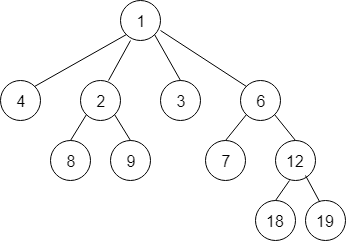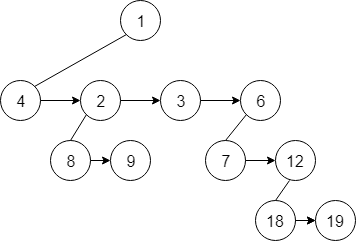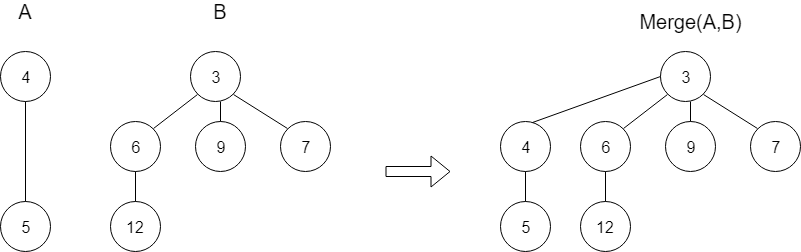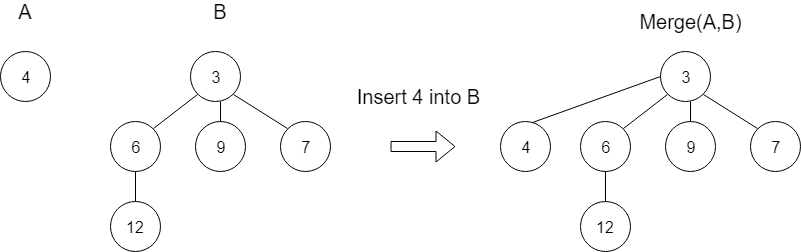×

Search anything:

# Pairing Heap

#### Data Structures heap pairing heapGet this book -> Problems on Array: For Interviews and Competitive Programming

Pairing heaps are a type of heap data structures which have fast running time for their operations. They are modificaton of Binomial Heap. Basically it is a type of self adjusting Binomial Heap which adjusts or rearrange themselves during the operations, due to which they remain balanced.

A pairing heap would either be an empty heap, or a pairing tree consisting of a root element and a list of pairing heaps(which may be empty). Pairing heaps maintain a min-heap property i.e. all parent nodes have smaller value than their children.Each Node consists following information:

1. A pointer to its leftmost child node
2. A pointer to its sibling nodes.
Following is the diagram which shows the pointers in pairing heap.### Basic Operations

1. Merge : Merge two pairing heaps.
2. Find Minimum : Find the minimum element from pairing heap
3. Insert : Insert a new element
4. Extract Minimum : Delete the minimum element from pairing heap

## Merge

Merge functions takes two pairing heap as input, A and B, and returns a pairing heap that contains all elements of A and B.

### Pseudocode

``````function merge(heap1, heap2)
if heap1 == Empty
return heap2
elsif heap2 == Empty
return heap1
elsif heap1.elem < heap2.elem
return Heap(heap1.elem, heap2 :: heap1.subheaps)
else
return Heap(heap2.elem, heap1 :: heap2.subheaps)
``````

Example:• If merge operation occurs between a non-empty heap and an empty heap, then merge function would just return that non-empty heap.
• And if both heaps are non-empty, then it would return a heap where the root of merged heap would be smallest root of two heaps.

## Find Minimum

Find Minimum function would return the minimum element of the heap.

Finding minimum element is pretty easy. We just need to get the top element of the heap.

### Pseudocode

``````function findMin(heap)
if heap == Empty
error
else
return heap.elem
``````

## Insertion

Insert function will insert an element in the existing pairing heap.
Merge Operation will be used for insertion operation.

### Pseudocode

``````function insert(elem, heap)
return merge(Heap(elem, []), heap)
``````
• Inserting an element is similar to merging the element in the heap, or merging two heaps(one of them have single element).## Extract Minimum

Basically, in a pairing heap(min-heap) the smallest element is it's root node. So for deletion of smallest element, deletion of root node should be done.

• If the root has two or more than two subtrees, then these subtress must be merged together into a singke tree after deletion of root node.
• But we don not have pointers to both the subtrees, so may be a deletion of minimum can cause violation in min-heap property. And to solve this we will use two-pass merge.
• Initially, two-pass pairing moves left to right merging pair of trees, and then on seconnd pass it moves right to left and merges the rightmost subtree with the remaining subtrees.

### Pseudocode

``````function extractMin(heap)
if heap == Empty
error
else
return merge-pairs(heap.subheaps)

function merge-pairs(l)
if length(l) == 0
return Empty
elsif length(l) == 1
return l
else
return merge(merge(l, l), merge-pairs(l[2.. ]))
``````

## Implementation

``````#include <iostream>
#include <cstdlib>
#include <vector>
using namespace std;

class PairNode {

int element;
PairNode *leftChild;
PairNode *nextSibling;
PairNode *prev;
PairNode(int element) {
this->element = element;
leftChild = NULL;
nextSibling = NULL;
prev = NULL;
}
};

class PairingHeap {

PairNode *root;
void compareAndLink(PairNode * &first, PairNode *second);
PairNode *combineSiblings(PairNode *firstSibling);
PairingHeap();
PairingHeap(PairingHeap &rhs);
bool isEmpty();
bool isFull();
int &findMin();
PairNode *Insert(int &x);
void deleteMin();
void deleteMin(int &minItem);
};

PairingHeap() {
root = NULL;
}

PairingHeap(PairingHeap & rhs) {
root = NULL;
*this = rhs;
}

PairNode Insert(int &x) {
PairNode *newNode = new PairNode(x);
if (root == NULL)
root = newNode;
else
return newNode;
}

int findMin() {
return root->element;
}

void deleteMin() {
PairNode *oldRoot = root;
if (root->leftChild == NULL)
root = NULL;
else
root = combineSiblings(root->leftChild);
delete oldRoot;
}

void deleteMin(int &minItem) {
if (isEmpty()) {
cout<<"The Heap is Empty"<<endl;
return;
}
minItem = findMin();
deleteMin();
cout<<"Minimum Element: "<<minItem<<" deleted"<<endl;
}

bool isEmpty() {
return root == NULL;
}

bool isFull() {
return false;
}

void compareAndLink(PairNode * &first, PairNode *second) {
if (second == NULL)
return;
if (second->element < first->element) {
second->prev = first->prev;
first->prev = second;
first->nextSibling = second->leftChild;
if (first->nextSibling != NULL)
first->nextSibling->prev = first;
second->leftChild = first;
first = second;
}
else {
second->prev = first;
first->nextSibling = second->nextSibling;
if (first->nextSibling != NULL)
first->nextSibling->prev = first;
second->nextSibling = first->leftChild;
if (second->nextSibling != NULL)
second->nextSibling->prev = second;
first->leftChild = second;
}
}

PairNode combineSiblings(PairNode *firstSibling) {
if (firstSibling->nextSibling == NULL)
return firstSibling;
static vector<PairNode *> treeArray(5);
int numSiblings = 0;
for (; firstSibling != NULL; numSiblings++)
{
if (numSiblings == treeArray.size())
treeArray.resize(numSiblings * 2);
treeArray[numSiblings] = firstSibling;
firstSibling->prev->nextSibling = NULL;
firstSibling = firstSibling->nextSibling;
}
if (numSiblings == treeArray.size())
treeArray.resize(numSiblings + 1);
treeArray[numSiblings] = NULL;
int i = 0;
for (; i + 1 < numSiblings; i += 2)
int j = i - 2;
if (j == numSiblings - 3)
for (; j >= 2; j -= 2)
return treeArray;
}

int main()
{
int choice, num, pos, minimumElement;
char ch;
PairingHeap h;
PairNode * pn;
while (1) {
cout << "1.Insert Element"<< endl;
cout << "2.Extract Minimum Element " << endl;
cout << "3.Find Minimum"<< endl;
cout << "4.Quit"<< endl;
cout << "Enter your choice : ";
cin >> choice;
switch(choice) {
case 1:
cout << "Enter the number to be inserted : ";
cin >> num;
pn = h.Insert(num);
break;
case 2:
h.deleteMin(num);
break;
case 3:
minimumElement = h.findMin();
cout << "Minimum Element is " << minimumElement << endl;
break;
case 4:
exit(1);
default:
cout<<"Wrong choice"<<endl;
}
}
return 0;
}
``````

## Time Complexity

1. Merge : O(log N)
2. Find Minimum : O(1)
3. Insertion : O(1)
4. Extract Minimum : O(log N)

## Applications

• Efficient implementation of prority queue.
• They are faster than binary heaps and Fibonacci heaps.
• Least Time Complexity in most of the operations.
• Can be used as an efficient way for implementing Dijsktra's Algorithm.#### Nisarg Shah

Intern at Electromech Corporation Company (2018), DataOne Innovation Labs (2019), OpenGenus (2019) and DataThinker (2019). B. Tech (2017 to 2021) in IT at Ahmedabad University